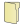Games
Problems
Go Pro!

# Logic

Pro Problems > Math > Logic

# Featured Pro ProblemsThree digits get together for brunch and discuss their relationshipsThe digits from zero to nine are competitors in a new reality TV show. Which digit will win?Prove that there is no two digit number which is the product of its digitsThere are infinitely many angles between 0 and 90 degrees for which sine and cosine values are both rational. Create a proof.Use a proof by induction to predict the value of the sum of the first n perfect squares.Prove by contradiction that the harmonic mean of two positive numbers lies between the two numbersDescribe the next picture in the sequence of pictures shown in the problemShow that the trig identity given is true for all non-negative integers n.# Full Directory Listing

NegationsLike us on Facebook to get updates about new resources